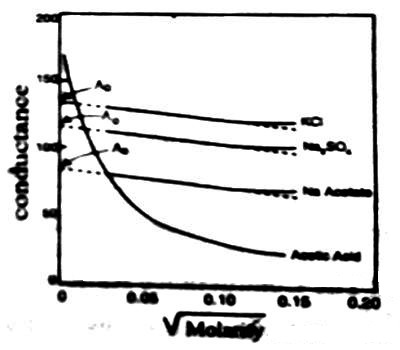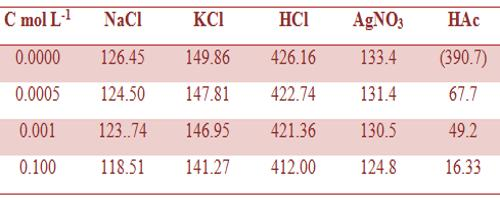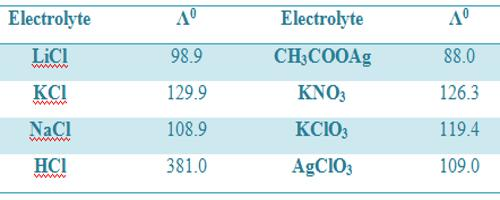Chemistry

# Conductance and Electrolyte ConcentrationConductance and Electrolyte Concentration

The conductivity of an electrolyte solution depends on concentration of the ionic species and behaves differently for strong and weak electrolytes. As the current is carried by ions the specific conductance will depend on the actual number of ions per unit volume of the solution. Hence the specific conductance progressively decreases with increasing dilution (i.e., decreasing concentration). However, the molar conductance, Λm increases with dilution and tends to attain a limiting constant value for strong electrolytes; but for weak electrolytes, like ethanoic acid, NH4OH etc., the molar conductance rises rapidly at low concentrations. The behaviors of both strong and weak electrolytes are shown in Figure.

The plots for Λm vs √molarity for strong electrolytes like KCI, Na2SO4, CH3COONa follow almost a linear relation at low concentration and the straight lines can be extrapolated to zero concentration to give Λ0m, the molar conductance at infinite dilution. But the curve for ethanoic acid shows a rapid upward trend for √c value approximately below 0.05. Extrapolation of such a curve to zero concentration is neither feasible nor at all reliable.Figure: Molar conductance vs square root of concentration

Table: Molar conductance (ohm-1 cm2 mol-1) of different electrolytes in aqueous solutions at 25°C.The measurement of Λm at very low concentrations serves as an excellent method for distinguishing between strong and weak electrolytes. Some data on molar conductance are given in Tables 1 and 2.

Table: Molar conductance (ohm-1 cm2 mol-1) at infinite dilution at 18°CIt is to be noted that values of Λ0m are different in different temperatures.